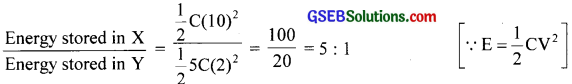# GSEB Solutions Class 12 Physics Chapter 2 Electrostatic Potential and Capacitance

Gujarat Board GSEB Textbook Solutions Class 12 Physics Chapter 2 Electrostatic Potential and Capacitance Textbook Questions and Answers, Additional Important Questions, Notes Pdf.

## Gujarat Board Textbook Solutions Class 12 Physics Chapter 2 Electrostatic Potential and Capacitance

### GSEB Class 12 Physics Electrostatic Potential and Capacitance Text Book Questions and AnswersQuestion 1.
Two charges 5 x 10-8 C and – 3 x 10-8 C are located 16 cm apart. At what point(s) on the line joining the two charges is the electric potential zero? Take the potential at infinity to be zero.
Solution:
On the line there are two locations where potential becomes zero.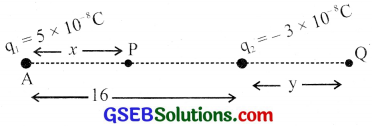For P,
$$\frac{5 \times 10^{-8}}{x}$$ = $$\frac{3 \times 10^{-8}}{16-x}$$
5( 16 – x) = 3x
80 – 5x = 3x
8x = 80
∴ x = 10cm
For Q,
$$\frac{5 \times 10^{-8}}{16+y}$$ = $$\frac{3 \times 10^{-8}}{y}$$
5y = 48 + 3y
∴ 2y = 48
y = 24 cm
i.e., at (16 + 24) = 40 cm from AQuestion 2.
A regular hexagon of side 10 cm has a charge 5μC at each of its vertices. Calculate the potential at the centre of the hexagon.
Solution:
If ‘q‘ is the charge, potential at ‘O’ is 9 x 109 x $$\frac { q }{ a }$$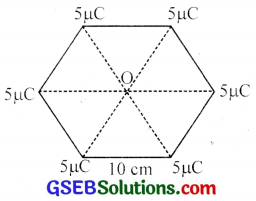where ‘a’ is the side of the hexagon.
∴ Total potential = $$\frac{6 \times q \times 9 \times 10^{9}}{a}$$
= $$\frac{9 \times 10^{9} \times 6 \times 5 \times 10^{-6}}{10 \times 10^{-2}}$$
= 2.7 x 106 V or 2.7 MV

Question 3.
Two charges 2 μC and -2 μC are placed at points A and B 6 cm apart.
(a) Identify an equipotential surface of the system.
(b) What is the direction of the electric field at every point on this surface?
Solution: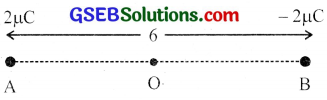(a) For the given system of the two charges, the equipotential surface will be a plane normal to the line AB joining the two charges and passing through its mid-point O. At any point on this plane, the potential is zero.

(b) The electric field is in a direction from point A to point B i.e. From the positive charge to negative charge and normal to the equipotential surface.

Question 4.
The spherical conductor of radius 12 cm has a charge of 1.6 x 10-7 C distributed uniformly on its surface. What is the electric field (a) inside the sphere, (b) just outside the sphere (c), at a point 18 cm from the centre of the sphere?
Solution:
R = 12 x 10-2 m, Q = 1.6 x 10-7C
(a) Zero

(b) E = $$\frac{9 \times 10^{9} \times 1.6 \times 10^{-7}}{\left(12 \times 10^{-2}\right)^{2}}$$
= $$\frac{9 \times 10^{9} \times 1.6 \times 10^{-7}}{12 \times 12 \times 10^{-4}}$$
= 105 NC-1

(c) Here R= 18 x 10-2m
Hence E = $$\frac{9 \times 10^{9} \times 1.6 \times 10^{-7}}{18 \times 18 \times 10^{-4}}$$
= $$\frac { 4 }{ 9 }$$ x 105 ≈ 4.4 x 104 NC-1Question 5.
A parallel plate capacitor with air between the plates has a capacitance of 8 pF (lpF = 10-12F). What will be the capacitance if the distance between the plates is reduced by half, and the space between them is filled with a substance of dielectric constant 6?
Solution:
C = 8pF,
C = $$\frac{\varepsilon_{0} \mathrm{~A}}{\mathrm{~d}}$$
C’ = $$\frac{\varepsilon_{\mathrm{r}} \varepsilon_{0} \mathrm{~A}}{\frac{\mathrm{d}}{2}}$$
= 2εr
∴ C’ = 2 x 6 x 8 x 10-12 F = 96 pF

Question 6.
Three capacitors each of capacitance 9 pF are connected in series.
(a) What is the total capacitance of the combination?
(b) What is the potential difference across each capacitor if the combination is connected to a 120 V supply?
Solution:
C = 9 pF
(a) Ceff = $$\frac { C }{ 3 }$$ = 3pF

(b) Potential difference = $$\frac { 120 }{ 3 }$$ = 40 V

Question 7.
Three capacitors of capacitances 2 pF, 3 pF and 4 pF are connected in parallel.
(a) What is the total capacitance of the combination?
(b) Determine the charge on each capacitor if the combination is connected to a 100V supply. Sol.
Solution;
C1 = 2pF, C2 = 3pF, C3 = 4pF
(a) 9 pF

(b) Q1 = 2 x 10-12 x 100 = 2 x 10-10C
Q2 = 3 x 10-10C
Q3 = 4 x 10-10C

Question 8.
In a parallel plate capacitor with air between the plates, each plate has an area of 6 x 10-3 m2 and the distance between the plates is 3 mm. Calculate the capacitance of the capacitor. If this capacitor is connected to a 100 V supply, what is the charge on each plate of the capacitor?
Solution:
A = 6 x 10-3 m2
d = 3 mm = 3 x 10-3m
C = $$\frac{\varepsilon_{0} \mathrm{~A}}{\mathrm{~d}}$$
= $$\frac{8.85 \times 10^{-12} \times 6 \times 10^{-3}}{3 \times 10^{-3}}$$
= 17.70 x 10-12
= 17.7 pF
Q = CV = 17.7 x 10-12 x 100
= 17.7 x 10-9C
= 1.77 nCQuestion 9.
Explain what would happen if in the capacitor given in Exercise.8, a 3 mm thick mica sheet (of dielectric constant = 6) were inserted between the plates,
(a) while the voltage supply remained connected.
(b) after the supply was disconnected.
Solution: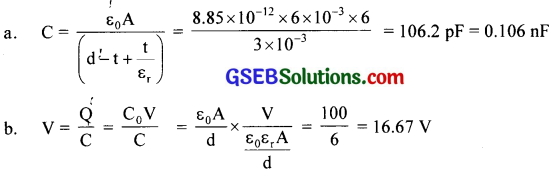Question 10.
A 12pF capacitor is connected to a 50V battery. How much electrostatic energy is stored in the capacitor?
Solution:
C = 12 pF, V = 50V
E = $$\frac { 1 }{ 2 }$$CV²
= $$\frac { 1 }{ 2 }$$ x 10-12 x 50 x 50
= 150 x 10-10
= 1.5 x 10-8 J

Question 11.
A 600pF capacitor is charged by a 200V supply. It is then disconnected from the supply and is connected to another uncharged 600 pF capacitor. How much electrostatic energy is lost in the process?
Solution:
C1 = 600 pF, V1 = 200V C2 = 600 pF, ∆E = ?
E1 = $$\frac { 1 }{ 2 }$$C1V1²
= $$\frac { 1 }{ 2 }$$ x 600 x 10-12 x 200 x 200
= 12 x 10-6J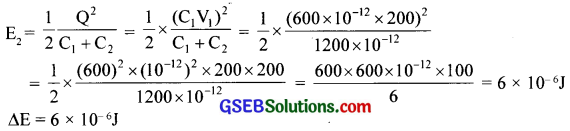Question 12.
A charge of 8 mC is located at the origin. Calculate the work done in taking a small charge of – 2 x 10-9 C from a point P (0, 0, 3 cm) to a point Q (0, 4 cm, 0), via a point R (0, 6 cm, 9 cm).
Solution:
q = 8 mC = 8 x 10-3C
q0 = – 2 x 10-9C
Work done = change in PE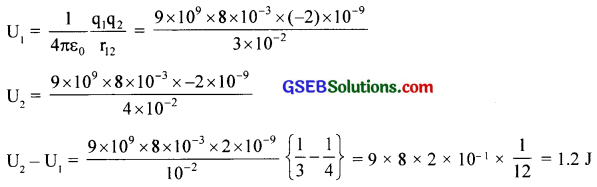Question 13.
A cube of side ‘b’ has a charge ‘q’ at each of its vertices. Determine the potential and electric field due to this charge array at the centre of the cube.
Solution:
$$\frac{\sqrt{b^{2}+2 b^{2}}}{2}$$
= $$\frac { b }{ 2 }$$$$\sqrt{3}$$ is the centre
Distance of centre of the cube from one vertex is $$\frac { b }{ 2 }$$$$\sqrt{3}$$.
Hence potential due to one charge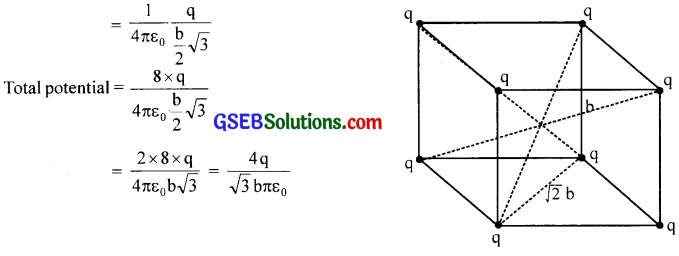Field = zeroQuestion 14.
Two tiny spheres carrying charges 1.5 μC and 2.5 μC are located 30 cm apart. Find the potential and electric field
(a) at the mid-point of the line joining the two charges, and
(b) at a point 10 cm from this midpoint in a plane normal to the line and passing through the mid-point.
q1 = 1.5 μ C
q2 = 2.5 μC
r = 30cm
(a) Potential at the midpoint = V1 + V2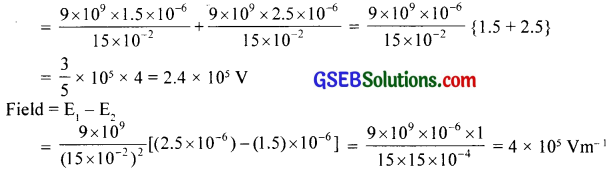(b)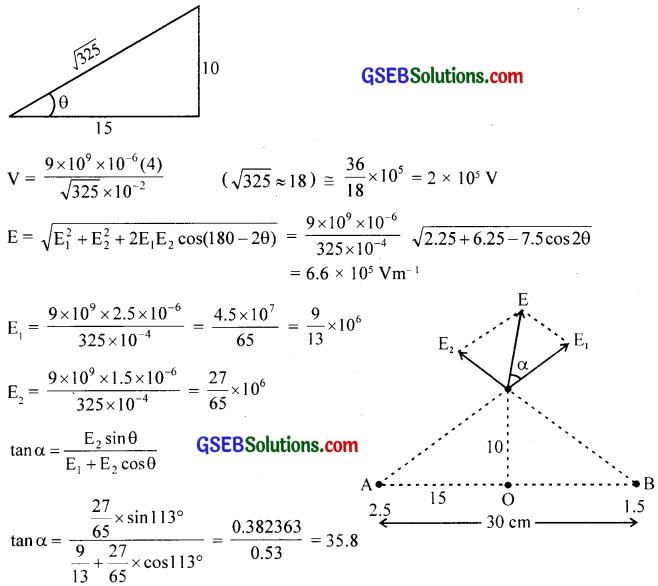∴ Inclination of resultant is 69.3° from AB

Question 15.
A spherical conducting shell of inner radius r1 and outer radius r2 has a charge Q.
(a) A charge ‘q’ is placed at the centre of the shell. What is the surface charge density on the inner and outer surfaces of the shell?
(b) Is the electric field inside a cavity (with no charge) zero, even if the shell is not spherical, but has any irregular shape? Explain.
Solution: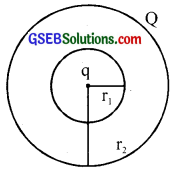(a) $$\frac{-q}{4 \pi r_{1}^{2}}$$, $$\frac{Q+q}{4 \pi r_{2}^{2}}$$
(b) Yes. By Gauss’ lawQuestion 16.
(a) Show that the normal component of electrostatic field has a discontinuity from one side of a charged surface to another given by (E2 – E1).$$\hat { n }$$ = $$\frac{\sigma}{\varepsilon_{0}}$$, where $$\hat { n }$$ is a unit vector normal to the surface at a point and σ is the surface charge density at that point. (The direction of n is from side 1 to side 2.) Hence show that just outside a conductor, the electric field is $$\frac{\sigma \hat{\mathbf{n}}}{\varepsilon_{0}}$$.
(b) Show that the tangential component of the electrostatic field is continuous from one side of a charged surface to another. [Hint: For (a), use Gauss law. For, (b) use the fact that work done by electrostatic field on a closed loop is zero.]
Solution:
(a) In figure, a Gaussian surface, in the form of a pill box is shown. It is of area ∆A (on end faces) and of negligible thickness. Let $$\overline { { E }_{ 1 } }$$ be the field below and $$\overline { { E }_{ 2 } }$$, the field above.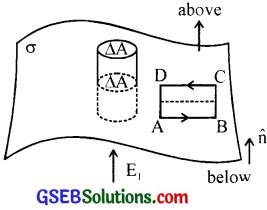The flux through upper surface = $$\overline { { E }_{ 2 } }$$ ∆$$\bar { A }$$
= ($$\overline { { E }_{ 2 } }$$.$$\hat { n }$$)∆A
Similarly flux through lower face = $$\overline { { E }_{ 1 } }$$ ∆$$\bar { A }$$
= (-$$\overline { { E }_{ 1 } }$$,.$$\hat { n }$$)∆A
Hence by Gauss theorem
( $$\overline { { E }_{ 2 } }$$ – $$\overline { { E }_{ 1 } }$$ ).$$\hat { n }$$∆$$\bar { A }$$
= $$\frac{\mathrm{q}}{\varepsilon_{0}}$$
= $$\frac{\sigma \Delta A}{\varepsilon_{0}}$$ ∴( $$\overline { { E }_{ 2 } }$$ – $$\overline { { E }_{ 1 }}$$ )$$\hat { n }$$ = $$\frac{\sigma}{\varepsilon_{0}}$$
Inside a conducting surface, E = 0 ∴$$\overline { { E }_{ 2 } }$$ = $$\bar { E }$$ = $$\frac{\sigma \hat{\mathbf{n}}}{\varepsilon_{0}}$$

(b) Consider a closed path ABCD. If E1n and E2n are the tangential components, then work done is E1n ∆l + E2n (-∆l) = 0 (property of electric field)
∴ E1n – E2n 0r E1n = E2n, showing that tangential components are continuous.

Question 17.
A long charged cylinder of linear charge density λ is surrounded by a hollow co-axial conducting cylinder. What is the electric field in the space between the two cylinders?
Solution:
Charge density = λ
The flux calculated for a cylindrical surface of radius r, over a length l is E.2πrl.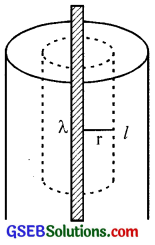By Gauss theorem, E.2πrl = $$\frac{l \lambda}{\varepsilon_{0}}$$
∴ E = $$\frac{\lambda}{2 \pi \varepsilon_{0}}$$
or
$$\bar { E }$$ = $$\frac{\lambda}{2 \pi r \varepsilon_{0}}$$

Question 18.
In a hydrogen atom, the electron and proton are bound at a distance of about 0.53 A.
(a) Estimate the potential energy of the system in eV, taking the zero of the potential energy at an infinite separation of the electron from the proton.
(b) What is the minimum work required to free the electron? Given that its kinetic energy in the orbit is half the magnitude of potential energy obtained in (a).
(c) What are the answers to (a) and (b) above if the zero of potential energy is taken at 1.06 A separation?
Solution:
(a) r = 0.53A =0.53 x 10-10m
Charge of electron = – e
Charge of proton = e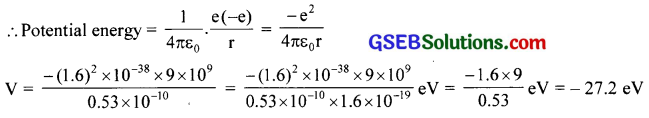(b) Total energy = -27.2 eV + 13.6 eV = -13.6 eV
The work required = 13.6 eV

(c) At 1.06 A distance
PE = -13.6 eV
It is brought to zero by adding + 13.6 eV
∴ PE at 0.53 is -13.6 eV and energy needed is 13.6 eV

Question 19.
If one of the two electrons of a H2 molecule is removed, we get a hydrogen molecular ion H2+. In the ground state of an H2+, the two protons are separated by roughly 1.5 A, and the electron is roughly 1 A from each proton. Determine the potential energy of the system. Specify your choice of zero potential energy.
Solution: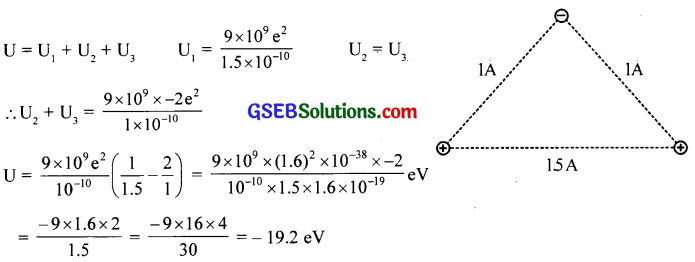Question 20.
Two charged conducting spheres of radii a and b are connected to each other by a wire. What is the ratio of electric fields at the surfaces of the two spheres? Use the result obtained to explain why the charge density on the sharp and pointed ends of a conductor is higher than on its flatter portions.
Solution:
With arbitrary charges q and Q for A and B respectively,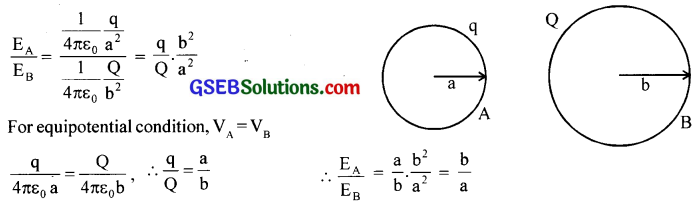E = $$\frac{\sigma}{\varepsilon_{0}}$$.
For pointed ends, σ = $$\frac { q }{ A }$$. As A → 0 E becomes extremely large. Flat means A → ∞ , hence E is very small.Question 21.
Two charges -q and +q are located at points (0, 0, -a) and (0, 0, a), respectively.
(a) What is the electrostatic potential at the points (0, 0, z) and (x, y, 0)?
(b) Obtain the dependence of potential on the distance r of a point from the origin when $$\frac { r }{ a }$$ >> 1.
(c) How much work is done in moving a small test charge from the point (5, 0, 0) to (-7, 0, 0) along the x-axis? Does the answer change if the path of the test charge between the same points is not along the x-axis?
Solution:
(a) The configuration is similar to a dipole.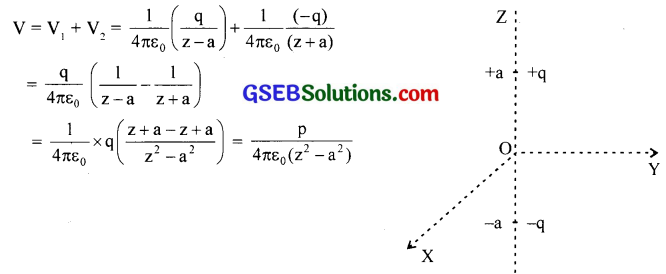In the case of (x, y, 0), it is the equatorial plane and hence potential is zero.

(b) The dependence on r is as $$\frac { 1 }{ { r }^{ 2 } }$$ type.

(c) Zero. No, because work done by electrostatic field between two points is independent of the path connecting the two points.

Question 22.
The figure shows a charge array known as an electric quadrupole. For a point on the axis of the quadrupole, obtain the dependence of potential on ‘r’ for $$\frac { r }{ a }$$ >> 1, and contrast your results with that due to an electric dipole, and an electric monopole (i.e., a single charge).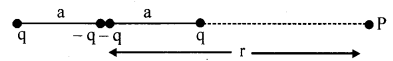Solution: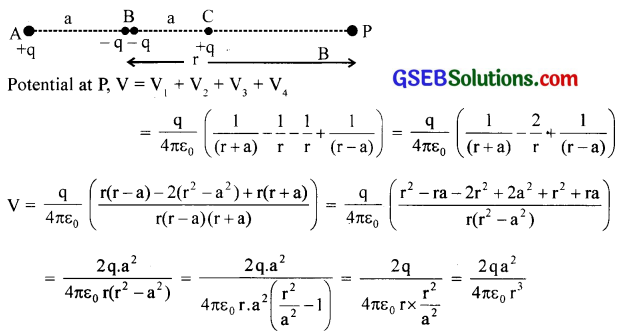V varies for quadrupole as $$\frac { 1 }{ { r }^{ 3 } }$$
V varies for dipole as $$\frac { 1 }{ { r }^{ 3 } }$$
V varies for monopole as $$\frac { 1 }{ r}$$

Question 23.
An electrical technician requires a capacitance of 2 μF in a circuit across a potential difference of 1 kV. A large number of 1 μF capacitors are available to him each of which can withstand a potential difference of not more than 400 V. Suggest a possible arrangement that requires the minimum number of capacitors.
Solution:
Ceff = 2μF = 2 x 10-6 F
V = 1 kV = 103V
C = 1 μF = 10-6F, rate, 400 V
Total change, Q = Ceff
V = 2 x 10-6 x 103
= 2 x 10-3C
Let there be ‘n’ capacitors in series and m such rows in the circuit.
Effective capacity, Ceff = $$\frac { C }{ n}$$ x m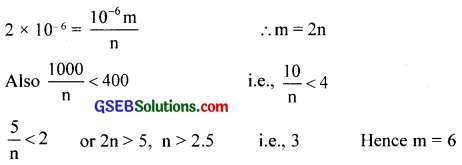Question 24.
What is the area of the plates of a 2F parallel plate capacitor, given that the separation between the plates is 0.5 cm? [You will realise from your answer why ordinary capacitors are in the range of pF or less. However, electrolytic capacitors do have a much larger capacitance (0.1F) because of very minute separation between the conductors.]
Solution:
A = ?, C = 2F, d = 0.5 x 10-2m
A = $$\frac{8.85 \times \mathrm{A} \times 10^{-12}}{0.5 \times 10^{-2}}$$
A = $$\frac{2 \times 10^{10}}{17.7}$$ = 1.13 x 109 m2 = 1.13 x 103 x (103)2 m2 = 1130 (km)2

Question 25.
Obtain the equivalent capacitance of the network in figure below. For a 300 V supply, determine the charge and voltage across each capacitor.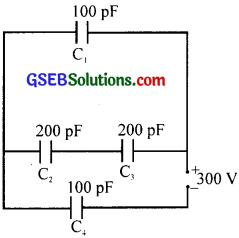Solution: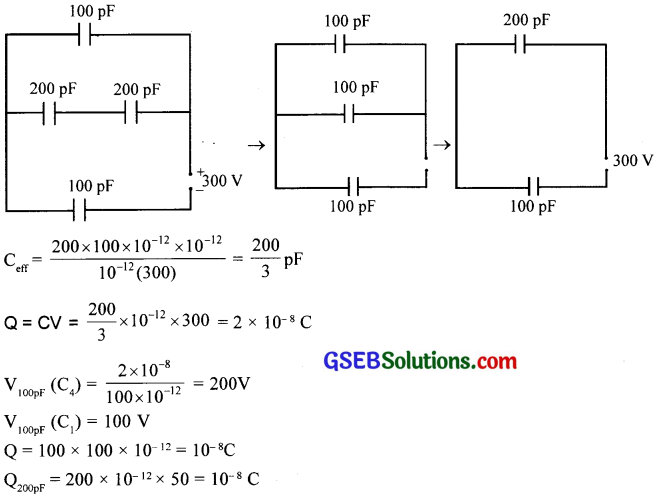Question 26.
The plates of a parallel plate capacitor have an area of 90 cm² each and are separated by 2.5 mm. The capacitor is charged by connecting it to a 400 V supply.
(a) How much electrostatic energy is stored by the capacitor?
(b) View this energy as stored in the electrostatic field between the plates, and obtain the energy per unit volume u. Hence arrive at a relation between u and the magnitude of electric field E between the plates.
Solution:
A = 90 cm² = 90 x 10-4
d = 2.5 mm = 2.5 x 10-3m
V = 400 V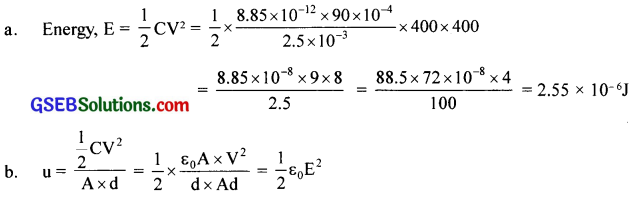Question 27.
A μ piF capacitor is charged by a 200 V supply. It is then disconnected from the supply and is connected to another uncharged 2 p.F capacitor. How much electrostatic energy of the first capacitor is lost in the form of heat and electromagnetic radiation?
Solution:
C1 = 4 x 10-6F
V = 200 V
C2 = 2 x 1o-6F
E1 = $$\frac { 1 }{ 2 }$$C1V2
= $$\frac { 1 }{ 2 }$$ x 4 x 10-6 x 200 x 200
= 8 x 1o-2
Q = C1V
= 4 x 10-6 x 200
= 8 x 10-4 C
E2 = $$\frac { 1 }{ 2 }$$$$\frac{\mathrm{Q}_{2}}{\mathrm{C}_{1}+\mathrm{C}_{2}}$$
= $$\frac { 1 }{ 2 }$$ x $$\frac{8 \times 10^{-4} \times 8 \times 10^{-2}}{6 \times 10^{-6}}$$
= $$\frac { 16 }{ 3 }$$ x 10-2
∴ ∆E = 8 x 10-2 – $$\frac { 16 }{ 3 }$$ x 10-2
= $$\frac { 8 }{ 3 }$$ x 10-2 JQuestion 28.
Show that the force on each plate of a parallel plate capacitor has a magnitude equal to $$\frac { 1 }{ 2 }$$ QE, where Q is the charge on the capacitor, and E is the magnitude of the electric field between the plates. Explain the origin of the factor $$\frac { 1 }{ 2 }$$.
Solution:
Force = $$\frac { 1 }{ 2 }$$ QE
Work done = $$\frac { 1 }{ 2 }$$ V x Q
The physical origin of the factor $$\frac { 1 }{ 2 }$$ in the force formula lies in the fact that just outside the conductor, the field is E, and inside it is zero. So the average value $$\frac { E }{ 2 }$$ contributes to the force.

Question 29.
A spherical capacitor has an inner sphere of radius 12 cm and an outer sphere of radius 13 cm. The outer sphere is earthed and the inner sphere is given a charge of 2.5 μC. The space between the concentric spheres is filled with a liquid of dielectric constant 32.
(a) Determine the capacitance of the capacitor.
(b) What is the potential of the inner sphere?
(c) Compare the capacitance of this capacitor with that of an isolated sphere of radius 12 cm. Explain why the latter is much smaller.
Solution: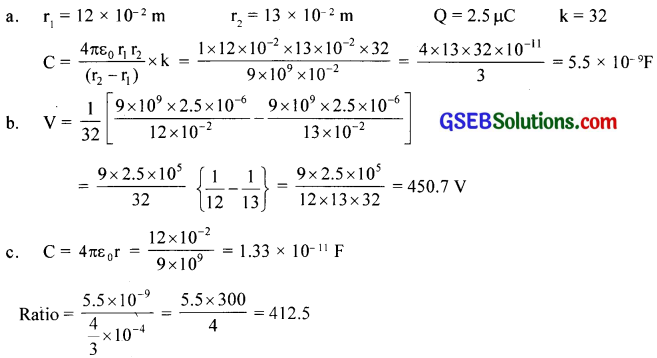Question 30.
(a) Two large conducting spheres carrying charges Q1 and Q1 are brought close to each other. Is the magnitude of electrostatic force between them exactly given by $$\frac{Q_{1} Q_{2}}{4 \pi \varepsilon_{0} r^{2}}$$, where r is the distance between their centres?
(b) If Coulomb’s law involved $$\frac { 1 }{ { r }^{ 3 } }$$ dependence (instead of $$\frac { 1 }{ { r }^{ 2 } }$$), would Gauss law be still true?
(c) A small test charge is released at rest at a point in an electrostatic field configuration. Will it travel along the field line passing through that point?
(d) What is the work done by the field of a nucleus in a complete circular orbit of the electron? What if the orbit is elliptical?
(e) We know that the electric field is discontinuous across the surface of a charged conductor. Is electric potential also discontinuous there?
(f) What meaning would you give to the capacitance of a single conductor?
(g) Guess a possible reason why water has a much greater dielectric constant (= 80) than say, mica (= 6).
Solution:
(a) No. The given relation is Coulomb’s law which is true for point charges. In the present case, as the spheres are brought closer, the distribution of charge on them becomes non­uniform.
(b) The surface area in space varies as r2 so that field varies as$$\frac { 1 }{ { r }^{ 2 } }$$. Hence $$\frac { 1 }{ { r }^{ 2 } }$$ dependence is essential.

(c) Not necessarily. The motion of charged particles need not be along the line of the field. It does so in the uniform field. The field gives the direction of acceleration and not that of velocity in general.

(d) Zero For any complete path in the electrostatic field (the shape does not matter), it is zero.

(e) No, Potential is continuous there.

(f) The single conductor can form a condenser with the other conductor at infinity. Hence the meaning of storage of charge retains.

(g) Water molecules are polar molecules.Question 31.
A cylindrical capacitor has two co-axial cylinders of length 15 cm and radii 1.5 cm and 1.4 cm. The outer cylinder is earthed and the inner cylinder is given a charge of 3.5μC. Determine the capacitance of the system and the potential of the inner cylinder. Neglect end effects (i.e., bending of field lines at the ends).
Solution:
l = 15 cm = 15 x 10-2m
a = 1.4 cm = 1.4 x 10-2m
b = 1.5 cm = 1.5 x 10-2 m
Q = 3.5 μC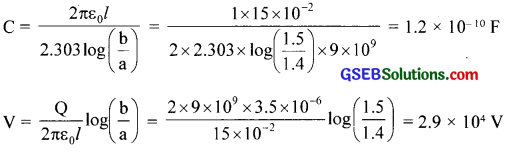Question 32.
A parallel plate capacitor is to be designed with a voltage rating of 1 kV, using a material of dielectric constant 3 and dielectric strength of about 107 Vm-1. (Dielectric strength is the maximum electric field a material can tolerate without breakdown, i.e., without starting to conduct electricity through partial ionisation.) For safety, we should like the field never to exceed, say 10% of the dielectric strength. What minimum area of the plates is required to have a capacitance of 50 pF?
Solution:
V= 103V
εr = 3
Edie = 107Vm-1
E = $$\frac { 10 }{ 100 }$$ x 107
= 106 Vm-1
A = ?, C = 50 pF = 50 x 10-12 F
E = $$\frac { V }{ d }$$ ∴ d = $$\frac { V }{ E }$$ = $$\frac { { 10 }^{ 3 } }{ { 10 }^{ 6 } }$$ = 10-3m
C = $$\frac{\varepsilon_{0} \varepsilon_{\mathrm{r}} \mathrm{A}}{\mathrm{d}}$$
∴A = $$\frac{C \cdot \mathrm{d}}{\varepsilon_{0} \varepsilon_{\mathrm{r}}}$$
= $$\frac{50 \times 10^{-12} \times 10^{-3}}{8.85 \times 10^{-12} \times 3}$$
= 1.9 x 10-3m2
= 19 x 10-4m2
= 19 cm2Question 33.
Describe schematically the equipotential surfaces corresponding to
(a) a constant electric Held in the z-direction,
(b) a field that uniformly increases in magnitude but remains in a constant (say, z) direction,
(c) a single positive charge at the origin, and
(d) a uniform grid consisting of long equally spaced parallel charged wires in a plane.
Solution:
(a) Equally spaced x-y planes.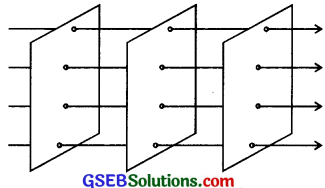(b) x-y planes getting closer and closer along the z-direction.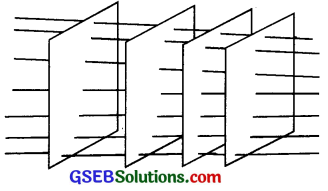(c) Concentric spherical surfaces gradually increasing in spacing.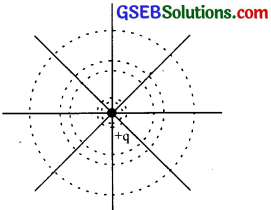(d) A periodically varying shape near the grid which gradually reaches the shape of the planes parallel to the grid at far distances.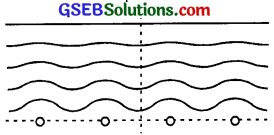Question 34.
In a Van de Graaff type generator, a spherical metal shell is to be a 15 x 106 V electrode. The dielectric strength of the gas surrounding the electrode is 5 x 107 Vm-1. What is the minimum radius of the spherical shell required? (You will learn from this exercise why one cannot build an electrostatic generator using a very small shell which requires a small charge to acquire a high potential.)
Solution:
V = 15 x 106 V
r = ?
E = $$\frac { V }{ r }$$
E = 5 x 107 Vm-1
r = $$\frac { V }{ E }$$
= $$\frac{15 \times 10^{6}}{5 \times 10^{7}}$$
= 0.3 m

Question 35.
A small sphere of radius r1 and charge q1 is enclosed by a spherical shell of radius r2 and charge q2. Show that if q1 is positive, the charge will necessarily flow from the sphere to the shell (when the two are connected by a wire) no matter what the charge q2 on the shell is.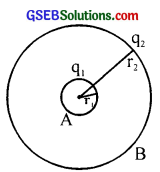Solution:
The potential inside the shell is constant and is equal to that on its surface. The potential difference then depends only on q1. Potential is highest on A. Hence charge flows from A to B irrespective of the value of q2.Question 36.
(a) The top of the atmosphere is at about 400 kV with respect to the surface of the earth, corresponding to an electric field that decreases with altitude. Near the surface of the earth, the field is about 100 Vm-1. Why then do we not get an electric shock as we step out of our house into the open? (Assume the house to be a steel cage so there is no field inside!)

(b) A man fixes outside his house one evening a two-meter high insulating slab carrying on its top a large aluminium sheet of area 1m2. Will he get an electric shock if he touches the metal sheet the next morning?

(c) The discharging current in the atmosphere due to the small conductivity of air is known to be 1800 A on average over the globe. Why then does the atmosphere not be discharge itself completely in due course and become electrically neutral? In other words, what keeps the atmosphere charged?

(d) What are the forms of energy into which the electrical energy of the atmosphere is dissipated during a bolt of lightning?

(Hint: The earth has an electric field of about 100 Vm-1 at its surface in the downward direction, corresponding to a surface charge density = -10-9 Cm-2. Due to the slight conductivity of the atmosphere upto about 50 km (beyond which it is a good conductor), about +1800 C is pumped every second into the earth as a whole. The earth, however, does not get dis-charged since thunderstorms and lightning occurring continually all over the globe pump an equal amount of negative charge on the earth.)
Solution:
(a) Our body and the ground form an equipotential surface. As we step out into the open, the original equipotential surfaces of open, the original equipotential surfaces of open-air change, keeping our head and the ground at the same potential.

(b) Yes. The steady discharging current in the atmosphere charges up the aluminium sheet gradually and raises its voltage to an extent depending on the capacitance of the capacitor.

(c) The atmosphere is continually being charged by thunderstorms and lightning all over the globe and discharged through regions of ordinary weather. The two opposing currents are, on average, in equilibrium.

(d) Light energy involved in lightning, heat, and sound energy in the accompanying thunder.

### GSEB Class 12 Physics Electrostatic Potential and Capacitance Additional Important Questions and Answers

Question 1.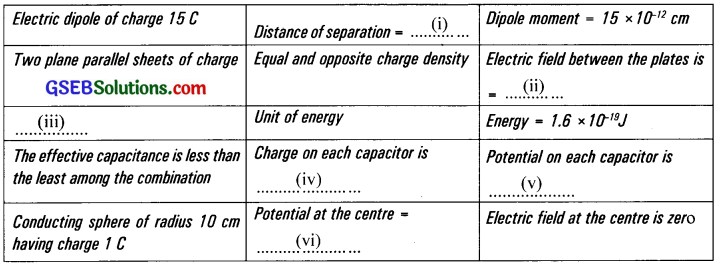(i) 1 x 10-6 m
(ii) Uniform
(iii) Electron Volt
(iv) Same
(v) Different
(vi) 9 x 10-4

Question 2.
Match the following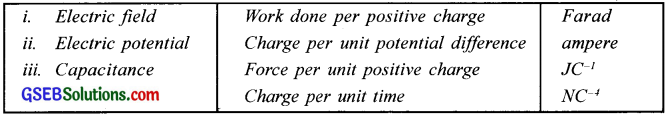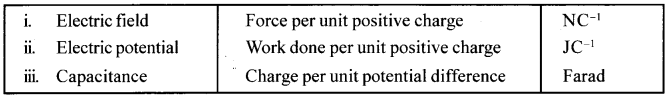Question 3.
What happens to a conductor when some charges are given to it?
Potential increasesQuestion 4.
(a) Are potential difference and potential energy the same?
(b) If they are not the same, then define them.
(c) Also state how they are related.
(a) No. The potential difference is a field property. Potential energy is the interaction energy of charge configuration.

(b) The electrostatic potential at a point in an electric field is defined as the work done in bringing a unit positive charge from infinity to that point against the field. The electric potential difference between the two points is equal to the work done in moving a unit test charge between them. The potential energy of a system of charges is defined as the work done in bringing the charges from infinity to form the system.

(c) Potential energy, U = Potential difference x Charge

Question 5.
A proton and an electron move between two points having a potential difference V.
(a) Which gains more energy?
(b) Define electron volt.
(c) How is 1 e V and 1 MeV related to joule?
(d) Write the dimensional formula of electrostatic potential.
(a) Both gain the same energy
Work done = energy gain = Potential difference x Charge
The p.d and charge are the same for each.

(b) One eV is the kinetic energy acquired by an electron accelerated on an electric field of p.d. one volt

(c) 1 MeV= 106 eV = 1.6 x 10-13 J
1 eV = 1.6 x 10-19 J

(d) [V] = [$$\frac { W }{ q }$$ ]
= $$\frac{\left[\mathrm{ML}^{2} \mathrm{~T}^{-2}\right]}{[\mathrm{I} \times \mathrm{T}]}$$
= M1L2T-3I-1]Question 6.
‘Work is done on the charge ’. What do you understand by this?
While moving the charge, work is to be done against repulsion of the field.

Question 7.
Three charges q1 q2 and q3 are placed in such a way that the distance between q1 and q2 is r12, q2 and q3 is r23, and q3 and q1 is r31.
(a) What is the potential energy of the system?
(b) In the above question if there are ‘N’point charges, then what is the net potential energy of the system? Give the expression.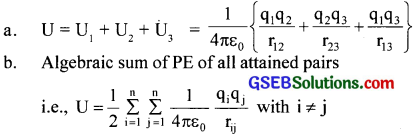Question 8.
You are given two graphs. What conclusion do you draw from the graphs?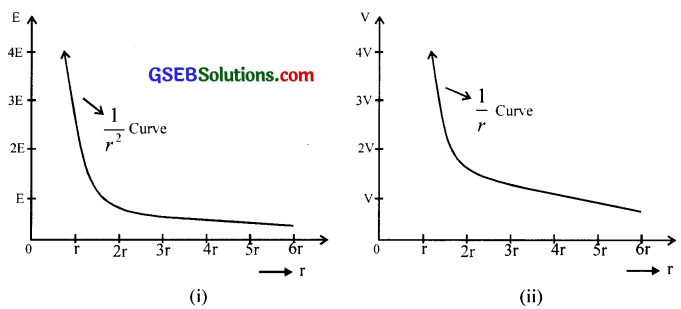The first figure shows the variation of field intensity of a point charge and the second figure shows the potential of a point charge.

Question 9.
A circle is drawn with centre as charge +q.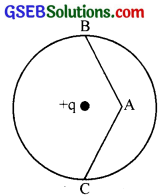(a) What is the work done in moving a charge +q from B to C along the circumference of the circle?
(b) If the charge +q is first taken from B to A and then from A to C, on which path work done will be more?
(a) No work is done. (Equipotential surface property)
(b) Work done will be the same. (p.d. is same irrespective of the path)

Question 10.
What is the potential difference between the surface and interior point of the charged conductor?
The potential at every point in a charged conductor is the same and is equal to the potential on the surface. Therefore the potential difference is zero.Question 11.
What happens when an uncharged conductor is placed near to a conductor?
Potential reduces by electrostatic induction.

Question 12.
What is the relation between potential difference and electric field intensity?
E = – $$\frac { dV }{ dr }$$

Question 13.
How can we increase the capacitance of a conductor?
By increasing the linear dimension.Question 14.
Potential of a conductor changes on charging.
(a) How is a charge related to the potential?
(b) What is the ratio called?
(a) On charging a body its potential rises. The potential of the body is proportional to the quantity of charge.

(b) V ∝ Q or V = $$\frac { Q }{ C }$$
∴C = $$\frac { Q }{ V }$$.
It is called capacity.

Question 15.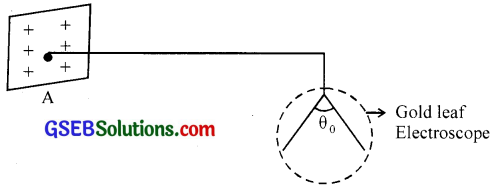In the above figure, an uncharged conducting plate B is brought near A (left side of A)

1. What will happen to plate B?
2. What will happen to the deflection θ0?
3. If plate B is earthed, what will happen to the potential and capacitance of plate A?

1. Induced charges appear in B
2. Effect of induced charge is to reduce the field of A, hence deflection of θ of the electroscope decreases.
3. Potential decreases and capacitance increases.Question 16.
In an experiment with a capacitor, the charge which was stored is measured for different values of changing p.d. The results are tabulated as follows: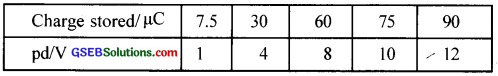(a) Plot a graph with a charge on Y-axis and p.d. on X-axis.
(b) Using the graph, calculate the capacitance of the capacitor.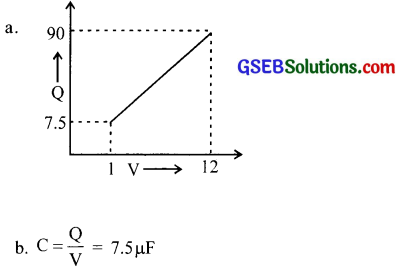Question 17.
pair of capacitors are connected in parallel while another identical pair in series. Which pair would be more dangerous to handle after being connected to the same voltage source?
The pair connected in parallel. The charge is higher can cause a high discharge current.

Question 18.
If p.d. across a capacitor is
(a) doubled
(b) halved, by what factor does the energy stored change in each case?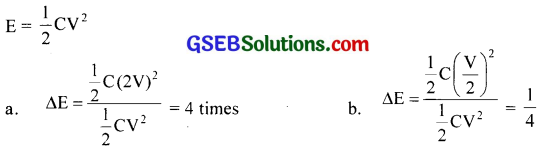Question 19.
If you were asked to design a capacitor would that be important in your design?
Area, separation, and dielectric constant.

Question 20.
In series combination, what is the charge on each plate of the capacitors?
SameQuestion 21.
How will the applied voltage be divided among the capacitors?
Directly proportional to capacity.

Question 22.
What is the work done in increasing the charge from Q1 to Q1 + δQ1, if the potential difference between the plates is V?
δw = V x δQ1

Question 23.
In which form and where is the energy of the capacitor stored?
Electrical energy and is stored between the plates in the form of the electric field.

Question 24.
What is the electric field in between the plates of a capacitor in the presence of a dielectric medium?
Decreases

Question 25.
A capacitor of capacitance C is connected to a voltage source of p.d. V. The capacitor gets charged.
(a) What happens to the voltage across the capacitor as the charge increases?
(b) What is the network done when the capacitor is fully charged?
(a) Increases

(b) W = $$\frac { 1 }{ 2 }$$CV2

Question 26.
What happens when a non-polar molecule is placed in an external electric field?
Electric polarisation takes place.Question 27.
What is the difference between a hydrogen molecule and an HCl molecule?
H2 is non-polar and HCl – polar

Question 28.
If the positive center of charge coincides with the negative centre of charge, what is its dipole moment?
zero

Question 29.
Classify the following molecules into
(a) Polar and non-polar. (H2O, H2, NH3, N2, CO2, CO, HCl, O2)
(b) Define polar and non-polar molecules.
(c) What is meant by dielectric polarization?
(a) Polar molecules: H2O, NH3, CO, HCl
Non-polar molecules: H2, N2, CO2, and O2

(b) polar and non-polar molecules:
A molecule is said to be polar if the centres of positive and negative charges do not coincide. That is. they are separated h a very short distance (oF the order 10-10m) and hence they form an electric dipole. Such a molecule then possesses a permanent dipole moment. In the absence of an electric field, these dipole moments are directed in random directions.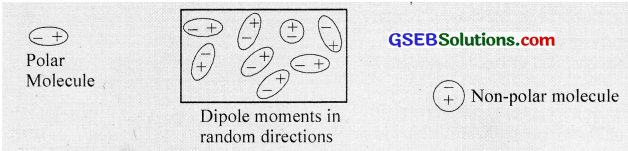Example H2O. HCl, NH3. CO etc.

A molecule is said to be non-polar if the centres of positive and negative charges coincide. So they cannot form an electric dipole and hence they have no permanent dipole moment. Example O2, N2, H2, CO2 etc.

In the presence of an electric field, the centres of gravity of positive and negative charges of a non-polar molecule get separated by a very short distance and form an electric dipole. Hence, the non-polar molecule gets a dipole moment b induction. The dipole moment so acquired is known as induced electric dipole moment. in the presence of an electric field, the dipoles get aligned parallel to the field and is said to be polarized and the phenomenon is known as electric polarization.

(c) A dielectric material, polar or non-polar, when placed in an electric field E0, the dipoles get aligned parallel to the field. The dielectric material set up a secondary electric field Ep of its own in a direction opposite to the applied field E0. This phenomenon is known as dielectric polarization. The net field strength gets reduced and is given as E = E0 – Ep.

Definition: The alignment of the dipole moment of permanent or induced dipoles of a dielec¬tric in an external electric field is called dielectric polarization.Question 30.
What is the electric field in between the plates of the capacitor, if ‘a ’ is the surface charge density?
E = $$\frac{\sigma}{\varepsilon_{0}}$$

Question 31.
A conducting spherical shell of radius R has a charge Q. A small sphere of radius r0 (r0 < R) carrying a charge q is introduced inside the large shell. What is the potential (i) at a point r (r > r0) due to the inner sphere
(ii) at a point r = R
(iii) at a point r = r0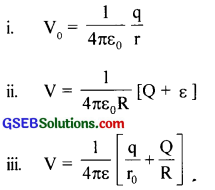Question 32.
Two copper spheres of radii, one hollow and the other solid are charged to the same potential.

1. Which of the two will hold more charge?
2. Can a metal sphere of radius 1 cm hold a charge of 1 C?

1. Both will hold the same charge
2. No
3. The capacity is smallQuestion 33.
Two capacitors when connected in series the effective capacity is 2 μF and when in parallel it is 9 μF. Calculate the value of each capacitor.
Let C1 and C2 be the capacity of the two capacitors.
Then, in series, Cs = $$\frac{\mathrm{C}_{1} \mathrm{C}_{2}}{\mathrm{C}_{1}+\mathrm{C}_{2}}$$
= 2 μF and in parallel, Cp = C1 + C2 = 9μF …. (1)
∴ C1C2 = 2(C1 + C2) = 2 x 9 = 18
C2 = $$\frac{18}{\mathrm{C}_{1}}$$
Substituting eq. (2) in eq. (1), we get, C1 = $$\frac{18}{\mathrm{C}_{1}}$$ = 9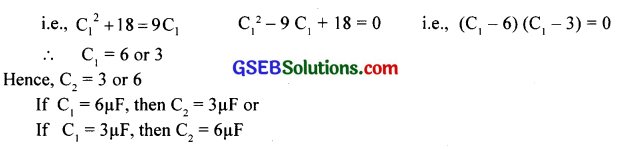Question 34.
What is the capacity of the capacitor having a plate area A of the figure given below?
Solution: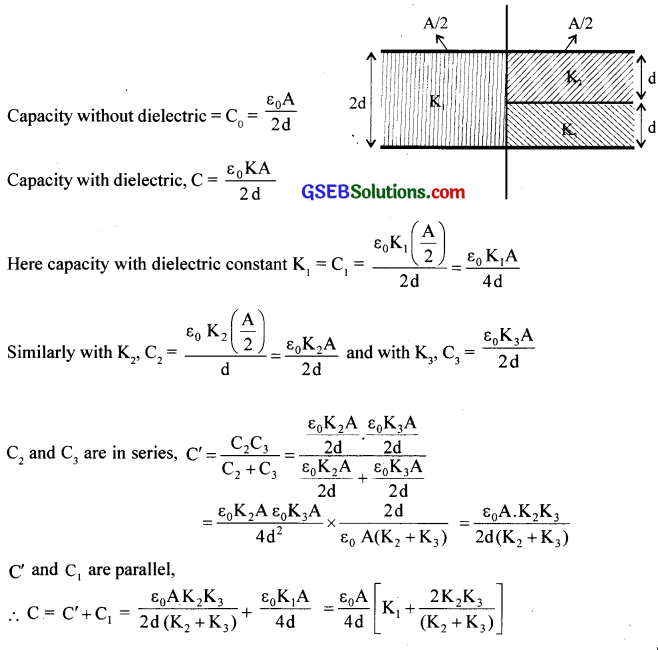Question 35.
Keeping the voltage of the charging source constant, what would be the percentage change in the energy stored in a parallel plate capacitor if the separation between its plates is decreased by 10%?
Energy stored in the capacitor is given by
E = $$\frac { 1 }{ 2 }$$CV2 and C =$$\frac{\varepsilon_{0} A}{d}$$, where d is the separation between the parallel plate capacitor.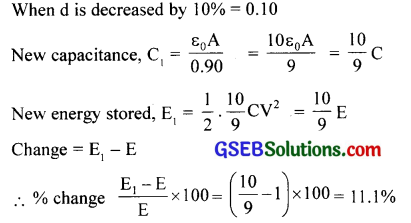Question 36.
Four-point charges are placed at the four corners of a square in the two ways (i) and (ii) as shown below. Will the
(i) electric field
(ii) electric potential, at the centre of the square, be the same or different in the two configurations and why?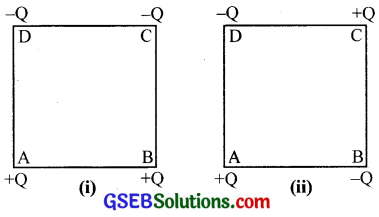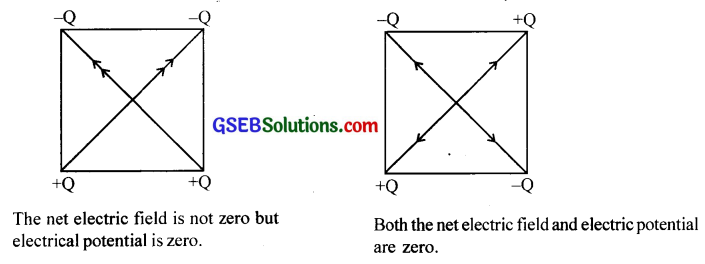Question 37.
How much work is done in moving a 500 pC charge between two points on an equipotential surface?
ZeroQuestion 38.
Two dielectric slabs of dielectric constants K1 and K2 are filled in between the two plates, each of area A of the parallel plate capacitor as shown in the figure. Find the net capacitance of the capacitor.
The arrangement is equivalent to a parallel combination of two capacitors, each with plate area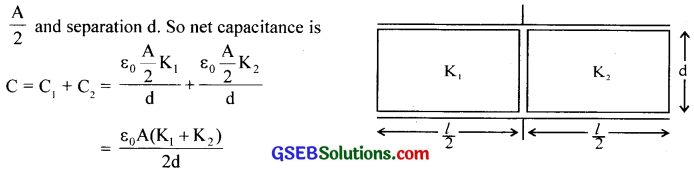Question 39.
An infinite number of charges, each of charge q, are located along the x-axis at x = 1, x = 2, x = 4, x = 8 and so on. Find the potential at x = 0.
Solution:
Potential at x = 0 due to other charges is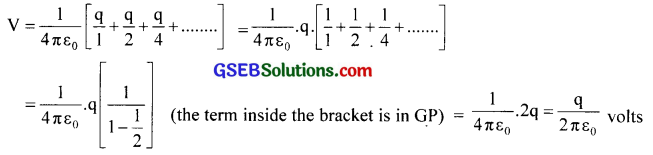Question 40.
Derive an expression for field intensity due to a uniformly charged ring at a point on the axis.
A ring of radius R is charged uniformly with linear charge density λ = $$\frac { dq }{ dl }$$. Consider a small element of length dl. The field intensity at the axial point P is dE = $$\frac{1}{4 \pi \varepsilon_{0}} \frac{\mathrm{dq}}{\mathrm{r}^{\prime 2}}$$. This can be resolved into dEsinθ – the perpendicular component and dEcosθ – the parallel component. The perpendicular component gets cancelled by symmetry. The total field is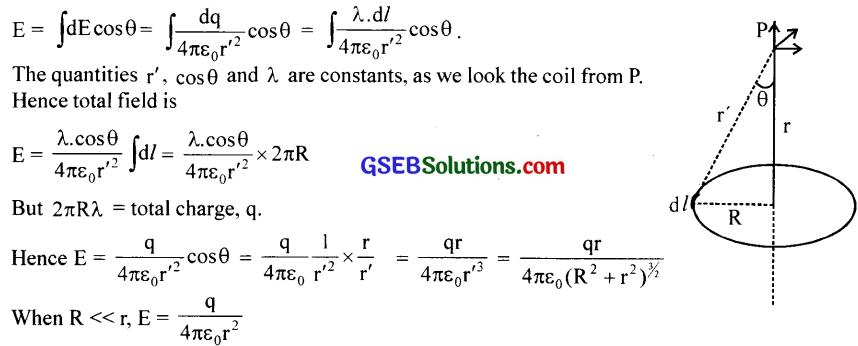Question 41.
X and Y are two parallel plate capacitors having the same area of plates and the same separation between the plates. X has air between the plates and Y contains a dielectric medium of εr = 5.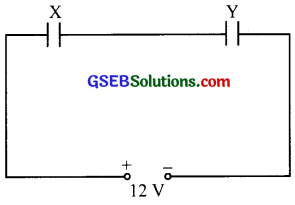Solution:
(i) Calculate the potential difference between the plates of X and Y.
(ii) What is the ratio of electrostatic energy stored in X and Y?
(i) Let V be the p.d. across X. Then p.d. across Y will be $$\frac { V }{ 5 }$$
∴ v + $$\frac { V }{ 5 }$$ = 12 volt ⇒ $$\frac { 6 V }{ 5 }$$ = 12 volt or V = 10 volt
p.d across Y = $$\frac { 10 }{ 5 }$$ = 2 volt
(ii)